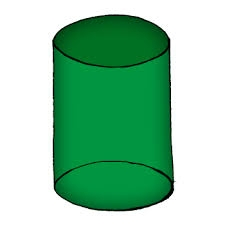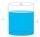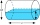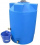# Collect rain water

The garden water tank has a cylindrical shape with a diameter of 80 cm and a height of 12 dm. How many liters of water will fit into the tank?

V =  603.2 l

### Step-by-step explanation:Did you find an error or inaccuracy? Feel free to write us. Thank you!Tips to related online calculators
Do you know the volume and unit volume, and want to convert volume units?
Do you want to round the number?

#### You need to know the following knowledge to solve this word math problem:

We encourage you to watch this tutorial video on this math problem:

## Related math problems and questions:

• Tank and waterWe poured a cylindrical tank with 3.5 liters of water. If the tank base diameter is 3 dm, how height is the water level in?
• ConvaHow many liters of water fit into the shape of a cylinder with a bottom diameter 20 cm and a height 45 cm?
• Water tankThe water tank has a cylindrical shape with a base diameter of 4.2 m and is 80 cm deep. How many minutes will take fill it 10 cm below the edge of the tank if water flowing 2 liters per second?
• The potDiameter of the pot 38 cm. The height is 30 cm. How many liters of water can fit in the pot?
• Water cylinderZuzana poured 785 ml of water into a measuring cylinder with a base radius of 5 cm. The water in the cylinder reached a height of 2 cm from the upper edge of the cylinder. How tall is the cylinder? (π = 3.14)
• CylinderThe cylinder-shaped container has 80 liters of water. The water reaches 45 cm height. How many water liters will be in a container if the water level extends to a height of 72 cm? Write the result in liters, write down only as a whole or decimal number.
• Water tankWhat is the height of the cuboid-shaped tank with the bottom dimensions of 80 cm and 50 cm if the 480 liters of water reaches 10 cm below the top?
• Liters in cylinderDetermine the height at which level 24 liters of water in a cylindrical container having a bottom diameter 36 cm.
• Mike builtMike built a cylindrical water tank with a radius of about 1.8 m and height of 2 meters. How many liters of water will it hold?
• Cylindrical tank9.6 hl of water is poured into a cylindrical tank with a bottom diameter of 1.2 m. What height in centimeters does the water reach?
• Water flow 2How many litres of water will flow in 7 minutes from a cylindrical pipe 1 cm in diameter, if the water flows at a speed of 30 km per hour
• Diameter of a cylinderThe diameter of the cylinder is 42 cm. How many times does the cylinder turn on a 66m long track?
• Water tankThe block-shaped tank is 2.5 m long, 100 cm wide and 12 dm high. In how many minutes will it be filled with water to two thirds if 40 liters of water flow into the tank per minute?
• Garden pondConcrete garden pond has bottom shape of a semicircle with a diameter 1.7 m and is 79 cm deep. Daddy wants make it surface. How many liters of water is in pond if watel level is 28 cm?
• Water levelHow high reaches the water in the cylindrical barell with a diameter of 12 cm if there is a liter of water? Express in cm with an accuracy of 1 decimal place.
• The cylindrical containerThe container has a cylindrical shape the base diameter 0.8 meters has a content area of the base is equal to the content area of the shell. How many full liters of water can be poured maximally into the container?
• Cylindrical tankCylindrical tank holds 600hl water and is deep 2.5 m. Calculate the diameter of the cylinder.